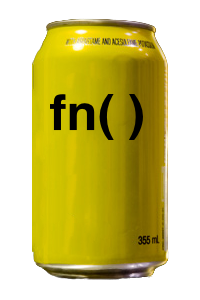# nofrillsLow-Cost Anonymous Functions

## Overview

nofrills is a lightweight R package that provides `fn()`, a more powerful variation of `function()` that:

• costs less — enables tidyverse quasiquotation so you don’t pay the price of functional impurity

• has the same great taste — supports a superset of `function()`’s syntax and capabilities

• is less filling

``fn(x, y = 1 ~ x + y)``

is equivalent to

``function(x, y = 1) x + y``

## Installation

``install.packages("nofrills")``

Alternatively, install the development version from GitHub:

``````# install.packages("devtools")
devtools::install_github("egnha/nofrills")``````

## Usage

### Same syntax as `function()` but shorter

``````fn(x ~ x + 1)
#> function (x)
#> x + 1

fn(x, y ~ x + y)
#> function (x, y)
#> x + y

fn(x, y = 2 ~ x + y)
#> function (x, y = 2)
#> x + y

fn(x, y = 1, ... ~ log(x + y, ...))
#> function (x, y = 1, ...)
#> log(x + y, ...)

# the only exception, cf. alist()
fn(x, ... = , y ~ log(x + y, ...))
#> function (x, ..., y)
#> log(x + y, ...)

fn(~ NA)
#> function ()
#> NA``````

### Supports quasiquotation

#### Unquote values

``````z <- 0

fn(x, y = !!z ~ x + y)
#> function (x, y = 0)
#> x + y

fn(x ~ x > !!z)
#> function (x)
#> x > 0``````

#### Unquote argument names

``````arg <- "y"

fn(x, !!arg := 0 ~ x + !!as.name(arg))
#> function (x, y = 0)
#> x + y``````

#### Splice in argument lists

``````args <- alist(x, y = 0)

fn(!!!args, ~ x + y)  # note the one-sided formula
#> function (x, y = 0)
#> x + y``````

#### Literally unquote with `QUQ()`, `QUQS()`

``````library(dplyr, warn.conflicts = FALSE)

summariser <- quote(mean)

my_summarise <- fn(df, ... ~ {
group_by <- quos(...)
df %>%
group_by(QUQS(group_by)) %>%
summarise(a = `!!`(summariser)(a))
})

my_summarise
#> function (df, ...)
#> {
#>     group_by <- quos(...)
#>     df %>% group_by(`!!!`(group_by)) %>% summarise(a = mean(a))
#> }``````

(Source: Programming with dplyr)

### Curry functions

#### Declare a curried function with `curry_fn()`

The syntax is the same as `fn()`. Using the literal unquoting operators `QUQ()`, `QUQS()`, you can “delay” unquoting to embed argument values in the innermost function:

``````compare_to <- curry_fn(target, x ~ identical(x, QUQ(target)))
is_this <- compare_to("this")

# The embedded value "this" renders the source comprehensible
is_this
#> function (x)
#> identical(x, "this")``````

#### Curry a function with `curry()`

``````curry(function(x, y, z = 0) x + y + z)
#> function (x)
#> function(y) function(z = 0) x + y + z

double <- curry(`*`)(2)
double(3)
#>  6``````

## Pure functions via quasiquotation

Functions in R are generally impure, i.e., the return value of a function will not in general be determined by the value of its inputs alone. This is because a function may depend on mutable objects in its lexical scope. Normally this isn’t an issue. But if you are working interactively and sourcing files into the global environment, say, or using a notebook interface (like Jupyter or R Notebook), it can be tricky to ensure that you haven’t unwittingly mutated an object that an earlier function depends upon.

• Consider the following function:

``````a <- 1
foo <- function(x) x + a``````

What is the value of `foo(1)`? It is not necessarily `2` because the value of `a` may have changed between the creation of `foo()` and the calling of `foo(1)`:

``````foo(1)
#>  2

a <- 0

foo(1)
#>  1``````

In other words, `foo()` is impure because the value of `foo(x)` depends not only on the value of `x` but also on the externally mutable value of `a`.

`fn()` enables you to write pure(r) functions by using quasiquotation to eliminate such indeterminacy.

• With `fn()`, you can unquote `a` to capture its value at the point of creation:

``````a <- 1
foo <- fn(x ~ x + !!a)``````

Now `foo()` is a pure function, unaffected by changes in its lexical scope:

``````foo(1)
#>  2

a <- 0

foo(1)
#>  2``````

## Alternatives to nofrills

Alternative anonymous-function constructors (which don’t support quasiquotation) include:

## Acknowledgement

The rlang package by Lionel Henry and Hadley Wickham makes nofrills possible. Crucially, rlang provides the engine for quasiquotation and expression capture.

## License

MIT Copyright © 2017–18 Eugene Ha